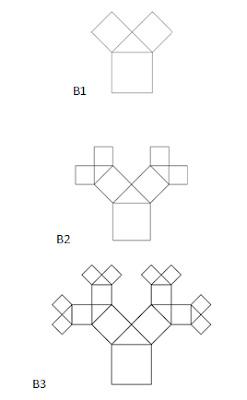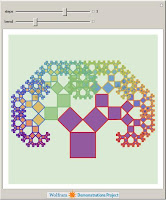## Monday, October 18, 2010

### "maverick mathematicians" and the power of grade school geometry

Earlier this week, the New York Times published this obituary for Benoît Mandelbrot, excerpted below:

Benoît B. Mandelbrot, a maverick mathematician who developed the field of fractal geometry and applied it to physics, biology, finance and many other fields, died on Thursday in Cambridge, Mass. He was 85. ....

Dr. Mandelbrot coined the term “fractal” to refer to a new class of mathematical shapes whose uneven contours could mimic the irregularities found in nature.

“Applied mathematics had been concentrating for a century on phenomena which were smooth, but many things were not like that: the more you blew them up with a microscope the more complexity you found,” said David Mumford, a professor of mathematics at Brown University. “He was one of the primary people who realized these were legitimate objects of study.”

In a seminal book, “The Fractal Geometry of Nature,” published in 1982, Dr. Mandelbrot defended mathematical objects that he said others had dismissed as “monstrous” and “pathological.” Using fractal geometry, he argued, the complex outlines of clouds and coastlines, once considered unmeasurable, could now “be approached in rigorous and vigorous quantitative fashion.”

For most of his career, Dr. Mandelbrot had a reputation as an outsider to the mathematical establishment. From his perch as a researcher for I.B.M. in New York, where he worked for decades before accepting a position at Yale University, he noticed patterns that other researchers may have overlooked in their own data, then often swooped in to collaborate.

...

Dr. Mandelbrot traced his work on fractals to a question he first encountered as a young researcher: how long is the coast of Britain? The answer, he was surprised to discover, depends on how closely one looks. On a map an island may appear smooth, but zooming in will reveal jagged edges that add up to a longer coast. Zooming in further will reveal even more coastline.

“Here is a question, a staple of grade-school geometry that, if you think about it, is impossible,” Dr. Mandelbrot told The New York Times earlier this year in an interview. “The length of the coastline, in a sense, is infinite.”

At last week's middle school math meet, we talked about a special kind of fractals, Pythagoras trees. The particular type of tree we investigated was made entirely out of squares and isosceles right triangles.

We started with the assumption that the biggest square in each fractal was 64, and tried to figure out how the area of the fractal grows as we add layers. One way to solve this problem is to use the Pythagorean theorem over and over and over again. That is the rather laborious approach that teachers came up with this summer at the Bard summer workshop for middle school math teachers.

Max Thomas of Hackett Middle School, however, came up with a very clever alternative approach. He noted that you can figure out that the isosceles right triangle in B1 must have area 16, because you can mentally "fold" it down into the big square below it, and easily confirm that it has area equal to one fourth of the big square. By similar reasoning, you can fold the same triangle in B1 over into the smaller squares in B1 and see that each of those two smaller squares must have an area of 32.

So, the total area of the fractal in B1 must be 64 + 16 + 32 +32 = 144. Can you extend Max's approach to figure out the areas of the succeeding fractals, and the general pattern as you add each layer of the fractals?You can also investigate other kinds of Pythagorean trees by downloading free interactive applications from Wolfram MathWorld here.Wolfram alpha also offers many other nice interactive fractal demonstrations here.

As Professor Mandelbrot and Max Thomas have both demonstrated, you don't always need to use high-powered mathematics to get very deep insights into fractal mathematics. Sometimes you don't even need the Pythagorean theorem, though playing with more complicated Pythagorean trees will certainly motivate more use and understanding of that idea. And if you persevere, the study of fractals can take you into more and more complex mathematics.

But first things first. Start with simple grade-school geometry and the Pythagorean theorem. See how far they can take you.

See what you can discover by investigating fractals playfully, starting with simple cases and working you way into the general patterns.